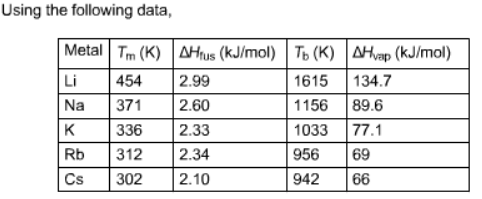# Problem: Calculate ΔSfus and ΔSvap for Na.

###### FREE Expert Solution

We’re being asked to calculate the ΔSfus and ΔSvap for Na.

We can calculate the entropy (ΔS) using the following equation:

$\overline{){\mathbf{∆}}{\mathbf{S}}{\mathbf{=}}\frac{\mathbf{∆}\mathbf{H}}{\mathbf{T}}}$

ΔS = entropy, J/mol•K
ΔH = enthalpy, J
T = temperature, K

93% (321 ratings)###### Problem Details

Calculate ΔSfus and ΔSvap for Na.